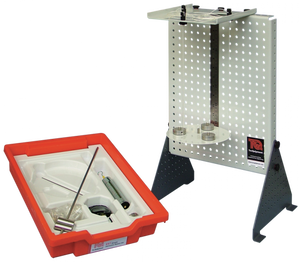ES07 - Simple Harmonic Motion

Regular price
\$750.00
Sale price
\$750.00

Description:

This versatile kit is part of a series that allows many experiments using different arrangements of their parts. Students, teachers or lecturers fit the parts of the kit to the Work Panel (ES1) (supplied separately) to study or show an engineering science topic.

This kit includes different pendulums and a spring to show students the principles and uses of simple harmonic motion.

Students test different pendulums and a spring to see how different factors, such as mass or pendulum length affect simple harmonic motion and the period of oscillation. The theory shows how to predict the period of oscillation for a given pendulum or spring for comparison with actual results. The kit includes an experiment with the Kater’s pendulum that shows the relationship between simple harmonic motion and gravity, for prediction of gravity to a reasonable accuracy.

The kit also has introduces students to a simple ‘spring rate’ test, and key scientific terms such as:

• Moments of inertia
• Parallel axis theorem.

TecQuipment supplies a CD-ROM with the Work Panel (ES1). It includes all the worksheets, guidance notes and lecturer notes (with answers) needed for typical experiments with each kit. The selection of parts in the kits and the choice of fixing points on the Work Panel means that teachers or lecturers may extend the experiments to an even greater range.

This versatile kit is part of a series that allows many experiments using different arrangements of their parts. Students, teachers or lecturers fit the parts of the kit to the Work Panel (ES1) (supplied separately) to study or show an engineering science topic.

This kit includes different pendulums and a spring to show students the principles and uses of simple harmonic motion.

Students test different pendulums and a spring to see how different factors, such as mass or pendulum length affect simple harmonic motion and the period of oscillation. The theory shows how to predict the period of oscillation for a given pendulum or spring for comparison with actual results. The kit includes an experiment with the Kater’s pendulum that shows the relationship between simple harmonic motion and gravity, for prediction of gravity to a reasonable accuracy.

The kit also has introduces students to a simple ‘spring rate’ test, and key scientific terms such as:

• Moments of inertia
• Parallel axis theorem.

TecQuipment supplies a CD-ROM with the Work Panel (ES1). It includes all the worksheets, guidance notes and lecturer notes (with answers) needed for typical experiments with each kit. The selection of parts in the kits and the choice of fixing points on the Work Panel means that teachers or lecturers may extend the experiments to an even greater range.

Learning Outcomes:

• Simple harmonic motion of simple, bifilar and trifilar pendulums of different lengths and masses
• Simple harmonic motion of a spring with different masses, and a simple spring rate test
• Simple harmonic motion of a compound pendulum
• Simple harmonic motion and gravity using a Kater’s pendulum

Curriculum Check:

VIC:

• Unit 3 - Area of Study 1 - Outcome 1

QLD:

• Unit 2 - Topic 2

WA:

• Unit 1
• Unit 2